# Viacheslav Borovitskiy (Slava)# Sequential Decision MakingGaussian processes — gold standard in uncertainty estimation

# Gaussian Processes

Definition. A Gaussian process is random function $f : X \times \Omega \to \R$ such that for any $x_1,..,x_n$, the vector $f(x_1),..,f(x_n)$ is multivariate Gaussian.

The distribution of a Gaussian process is characterized by

• a mean function $m(x) = \E(f(x))$,
• a kernel (covariance) function $k(x, x') = \Cov(f(x), f(x'))$,

Notation: $f \~ \f{GP}(m, k)$.

The kernel $k$ must be positive (semi-)definite.

# Gaussian Process Regression

Takes

• a prior Gaussian Process $\f{GP}(m, k)$
• and data $(x_1, y_1), .., (x_n, y_n) \in X \x \R$,

giving the posterior Gaussian process $\f{GP}(\hat{m}, \hat{k})$.

The functions $\hat{m}$ and $\hat{k}$ may be explicitly expressed in terms of $m$ and $k$.

# An Application: Bayesian Optimization

Goal: minimize unknown function $\phi$ in as few evaluations as possible.

1. Build GP posterior $f \given \v{y}$ using data $(x_1,\phi(x_1)),..,(x_n,\phi(x_n))$.
2. Choose $$\htmlClass{fragment}{ x_{n+1} = \argmax_{x\in\c{X}} \underbrace{\alpha_{f\given\v{y}}(x).}_{\text{acquisition function}} }$$ For instance, expected improvement $$\alpha_{f\given\v{y}}(x) = \E_{f\given\v{y}} \max(0, {\displaystyle\min_{i=1,..,n}} \phi(x_i) - f(x)).$$

# Bayesian Optimization# Applications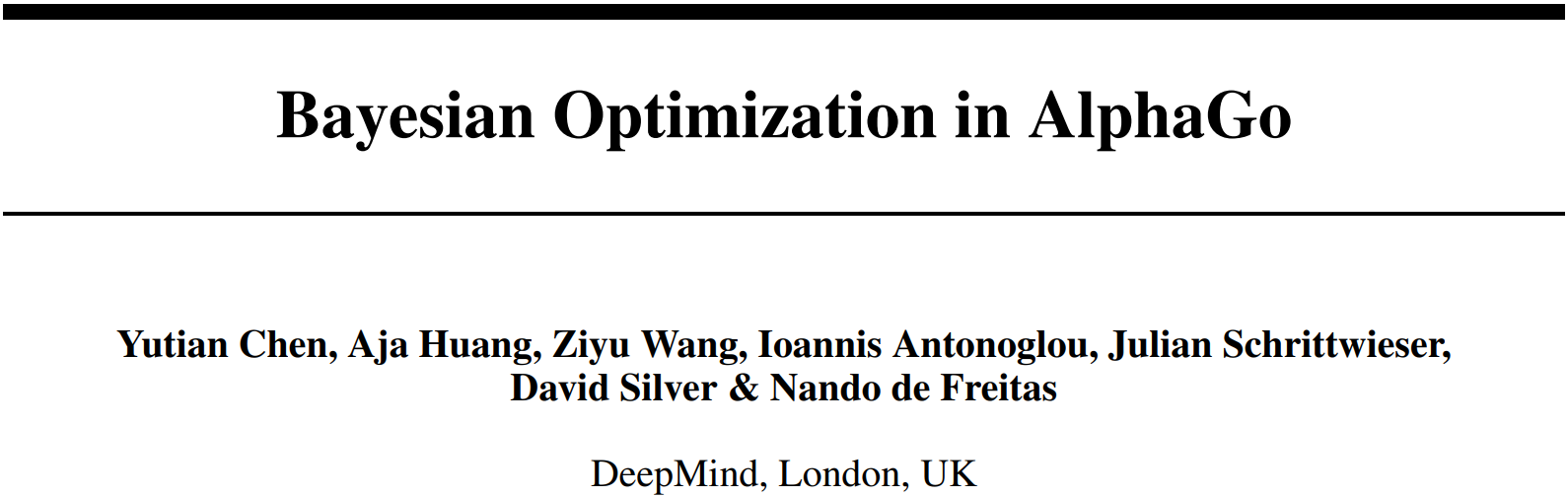Also

• geostatistics,
• robotics,
• more...

# Algorithms Connected to Gaussian Processes

ConditioningSamplingAnd hyperparameter optimization! Complexity: $O(N^3 + M^3)$ for $N$ data points and $M$ sampling nodes.

# Efficient Conditioning

Idea. Approximate the posterior process $\f{GP}(\hat{m}, \hat{k})$ with a simpler process belonging to some parametric family $\f{GP}(m_{\theta}, k_{\theta})$.

$O(N^3)$ turns into $O(N K^2)$ where $K$ controls accuracy

# Efficient Sampling From Priors

Priors are usually assumed stationary.

Idea. Approximate with a process of form

$$\htmlData{class=yoyo}{ \tilde{f}(x) = \sum_{l=1}^L w_l \phi_l(x) \qquad w_l \overset{\textrm{i.i.d.}}{\sim} N(0, 1), }$$

which is easy to sample from.

$O(M^3)$ turns into $O(M L)$ where $L$ controls accuracy

# Efficient Sampling From Posteriors

Idea. From a parametric family $\f{GP}(m_{\theta}, k_{\theta})$ build another family $\f{GP}(\tilde{m}_{\theta}, \tilde{k}_{\theta})$ which is easy to sample from.

$O(N^3 + M^3)$ turns into $O(N K^2 + M L)$ where $K$ and $L$ control accuracy

# Euclidean Priors: Matérn Gaussian Processes

$$\htmlData{class=fragment fade-out,fragment-index=9}{ \footnotesize \mathclap{ k_{\nu, \kappa, \sigma^2}(x,x') = \sigma^2 \frac{2^{1-\nu}}{\Gamma(\nu)} \del{\sqrt{2\nu} \frac{\norm{x-x'}}{\kappa}}^\nu K_\nu \del{\sqrt{2\nu} \frac{\norm{x-x'}}{\kappa}} } } \htmlData{class=fragment d-print-none,fragment-index=9}{ \footnotesize \mathclap{ k_{\infty, \kappa, \sigma^2}(x,x') = \sigma^2 \exp\del{-\frac{\norm{x-x'}^2}{2\kappa^2}} } }$$ $\sigma^2$: variance $\kappa$: length scale $\nu$: smoothness
$\nu\to\infty$: Gaussian kernel (RBF)$\nu = 1/2$$\nu = 3/2$$\nu = 5/2$$\nu = \infty$

# Non-Euclidean Domains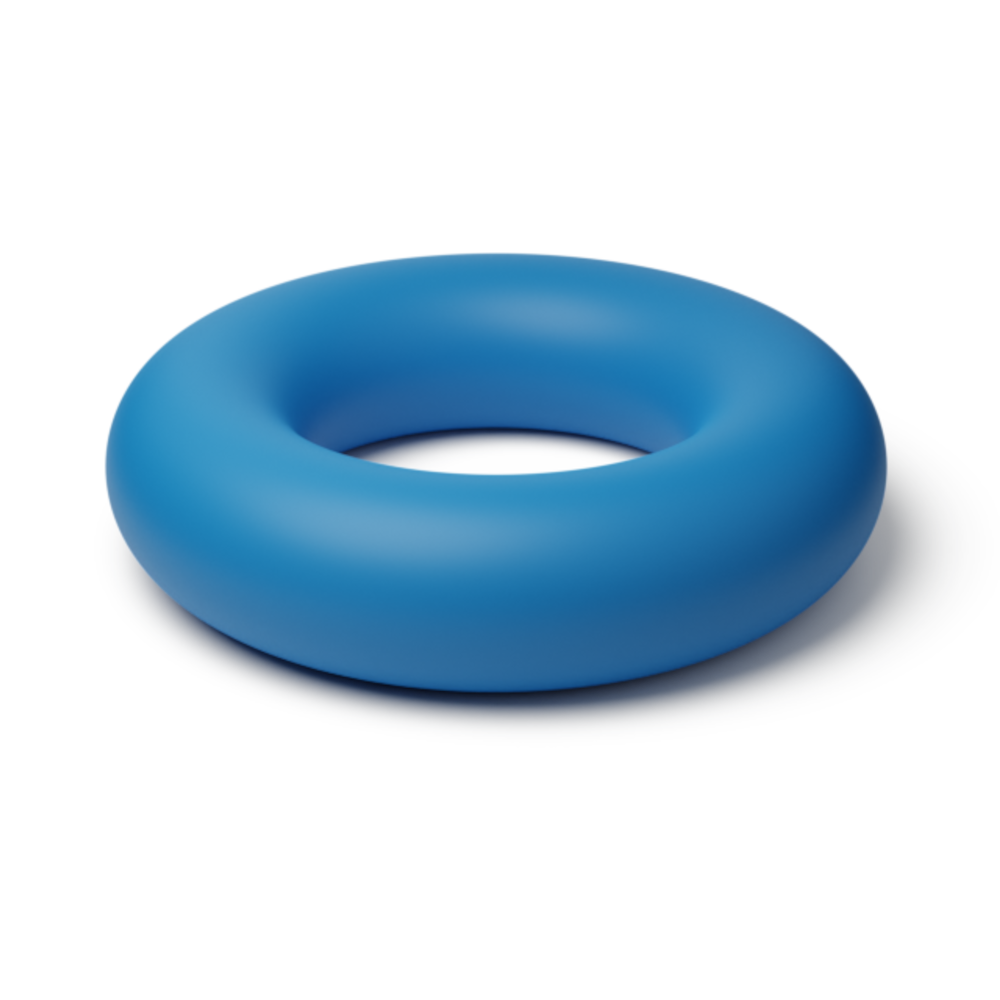### Manifolds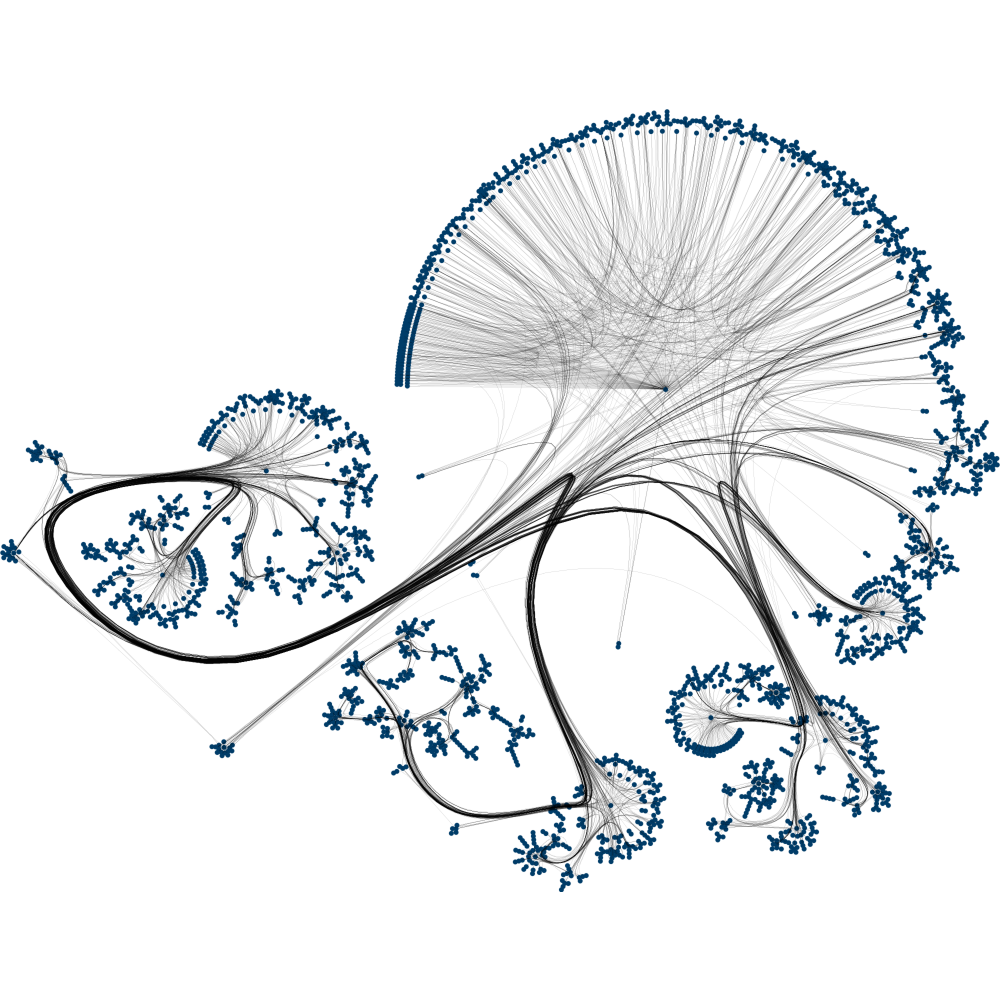# Explicit Formula for Compact Riemannian Manifolds

$$k_{\nu, \kappa, \sigma^2}(x,x') = \frac{\sigma^2}{C_\nu} \sum_{n=0}^\infty \del{\frac{2\nu}{\kappa^2} - \lambda_n}^{-\nu-\frac{d}{2}} f_n(x) f_n(x')$$

## $\lambda_n, f_n$: eigenvalues and eigenfunctions of the Laplace–Beltrami

Examples: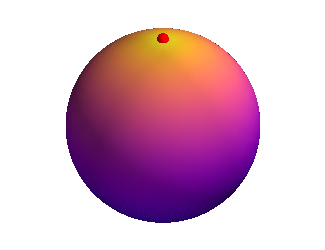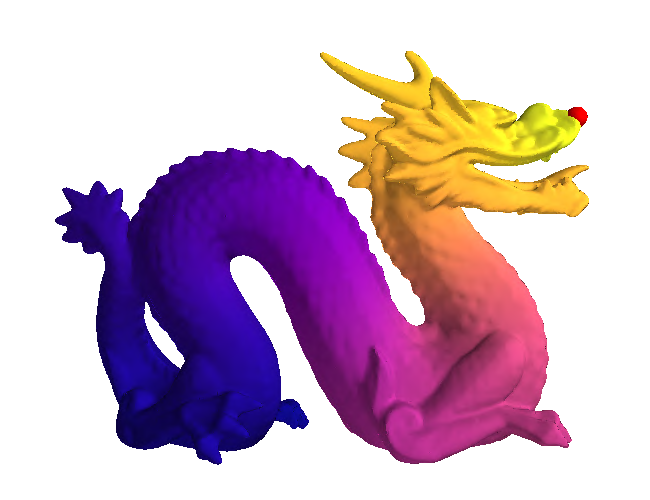# Explicit Formula for Finite Undirected Graphs

$$k_{\nu, \kappa, \sigma^2}(i, j) = \frac{\sigma^2}{C_{\nu}} \sum_{n=0}^{\abs{V}-1} \del{\frac{2\nu}{\kappa^2} + \mathbf{\lambda_n}}^{-\nu} \mathbf{f_n}(i)\mathbf{f_n}(j)$$

## $\lambda_n, \mathbf{f_n}$: eigenvalues and eigenvectors of the Laplacian matrix

Examples:# Graph Matérn kernels: examples# An Application: Modeling Dynamical Systems with Uncertainty

$$\htmlData{fragment-index=0,class=fragment}{ x_0 } \qquad \htmlData{fragment-index=1,class=fragment}{ x_1 = x_0 + f(x_0)\Delta t } \qquad \htmlData{fragment-index=2,class=fragment}{ x_2 = x_1 + f(x_1)\Delta t } \qquad \htmlData{fragment-index=3,class=fragment}{ .. }$$

# Pendulum Dynamics: GP on a cylinder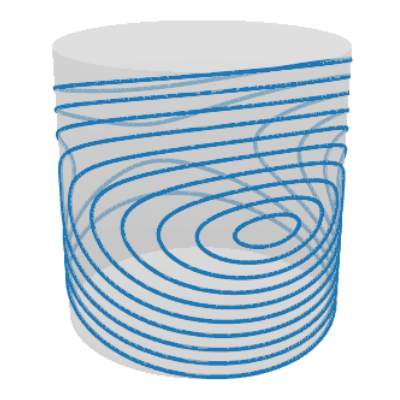### (a) Ground truth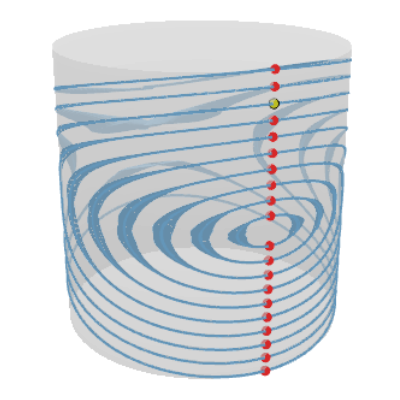# Traffic Speed Extrapolation on a Road Network### (a) Prediction# Wind Speed Modeling: Vector Fields on Manifolds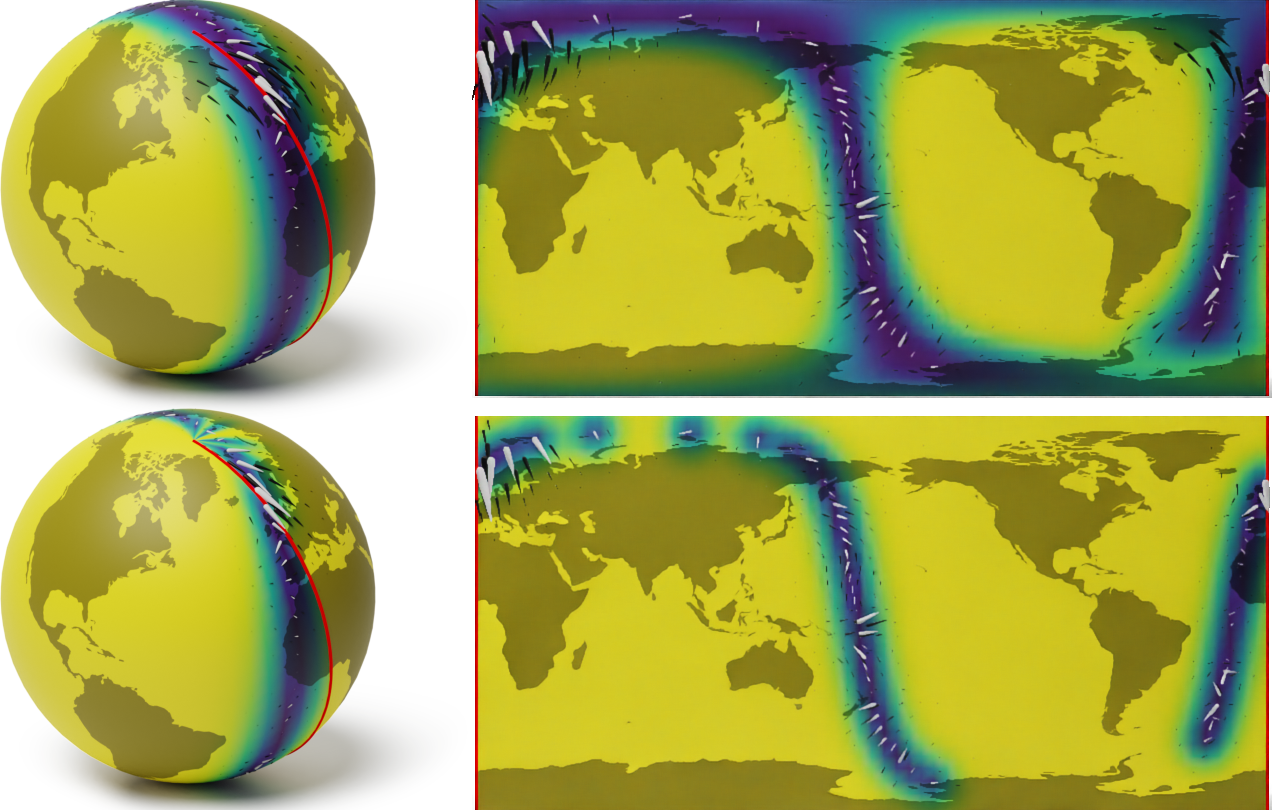# Thank you!

Special thanks: Ekaterina Noskova for the wonderful Bayesian optimization animations. viacheslav.borovitskiy@gmail.com                       https://vab.im# References

J. Wilson, V. Borovitskiy, A. Terenin, P. Mostowsky, M. P. Deisenroth. Efficiently sampling functions from Gaussian process posteriors. International Conference on Machine Learning, 2020.

V. Borovitskiy, A. Terenin, P. Mostowsky, M. P. Deisenroth. Matérn Gaussian Processes on Riemannian Manifolds.
In Neural Information Processing Systems (NeurIPS) 2020.

V. Borovitskiy, I. Azangulov, A. Terenin, P. Mostowsky, M. P. Deisenroth. Matérn Gaussian Processes on Graphs.
In International Conference on Artificial Intelligence and Statistics (AISTATS) 2021.

J. Wilson, V. Borovitskiy, A. Terenin, P. Mostowsky, M. P. Deisenroth. Pathwise Conditioning of Gaussian Processes. Journal of Machine Learning Research, 2021.

N. Jaquier, V. Borovitskiy, A. Smolensky, A. Terenin, T. Asfour, L. Rozo. Geometry-aware Bayesian Optimization in Robotics using Riemannian Matérn Kernels. To appear in Conference on Robot Learning (CoRL), 2021.

M. Hutchinson, A. Terenin, V. Borovitskiy, S. Takao, Y. W. Teh, M. P. Deisenroth. Vector-valued Gaussian Processes on Riemannian Manifolds via Gauge-Independent Projected Kernels. To appear in NeurIPS 2021.

viacheslav.borovitskiy@gmail.com                       https://vab.im